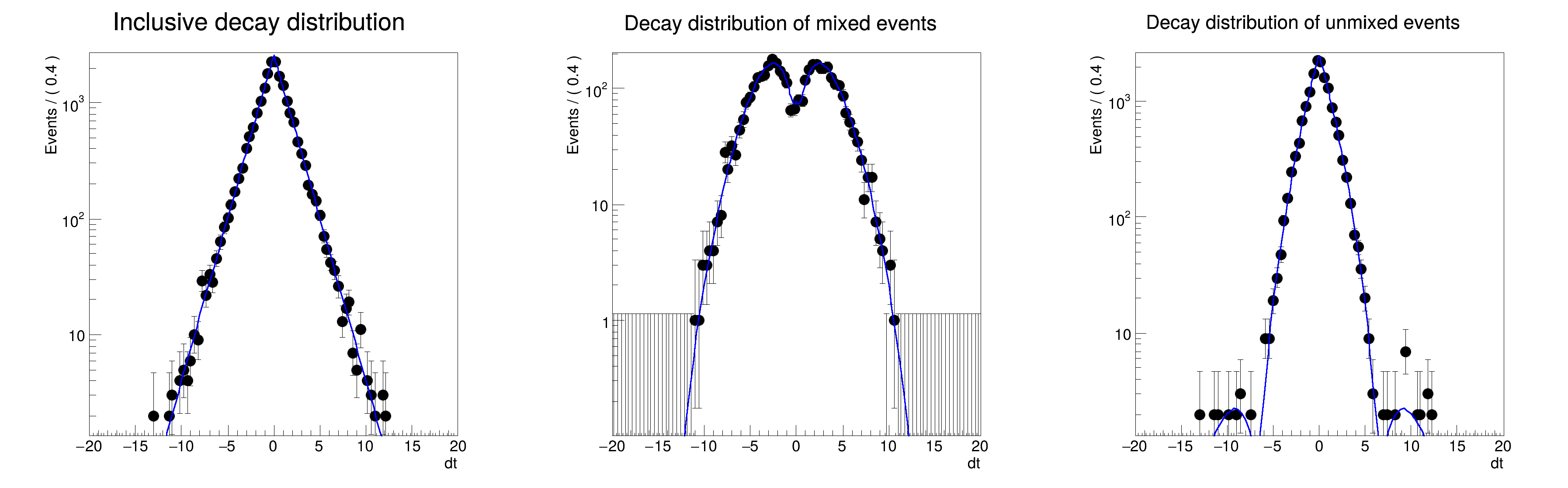ROOT   Reference Guiderf310_sliceplot.C File Reference

## Detailed DescriptionMultidimensional models: projecting pdf and data slices in discrete observables[#1] INFO:Plotting -- RooAbsReal::plotOn(bmix) plot on dt integrates over variables (tagFlav,mixState)
[#1] INFO:Plotting -- RooTreeData::plotOn: plotting 3787 events out of 20000 total events
[#1] INFO:Plotting -- RooAbsReal::plotOn(bmix) plot on dt represents a slice in (mixState)
[#1] INFO:Plotting -- RooAbsReal::plotOn(bmix) plot on dt integrates over variables (tagFlav)
[#1] INFO:Plotting -- RooTreeData::plotOn: plotting 16213 events out of 20000 total events
[#1] INFO:Plotting -- RooAbsReal::plotOn(bmix) plot on dt represents a slice in (mixState)
[#1] INFO:Plotting -- RooAbsReal::plotOn(bmix) plot on dt integrates over variables (tagFlav)
#include "RooRealVar.h"
#include "RooDataSet.h"
#include "RooGaussModel.h"
#include "RooDecay.h"
#include "RooBMixDecay.h"
#include "RooCategory.h"
#include "TCanvas.h"
#include "TAxis.h"
#include "RooPlot.h"
using namespace RooFit;
{
// C r e a t e B d e c a y p d f w it h m i x i n g
// ----------------------------------------------------------
// Decay time observables
RooRealVar dt("dt", "dt", -20, 20);
// Discrete observables mixState (B0tag==B0reco?) and tagFlav (B0tag==B0(bar)?)
RooCategory mixState("mixState", "B0/B0bar mixing state");
RooCategory tagFlav("tagFlav", "Flavour of the tagged B0");
// Define state labels of discrete observables
mixState.defineType("mixed", -1);
mixState.defineType("unmixed", 1);
tagFlav.defineType("B0", 1);
tagFlav.defineType("B0bar", -1);
// Model parameters
RooRealVar dm("dm", "delta m(B)", 0.472, 0., 1.0);
RooRealVar tau("tau", "B0 decay time", 1.547, 1.0, 2.0);
RooRealVar w("w", "Flavor Mistag rate", 0.03, 0.0, 1.0);
RooRealVar dw("dw", "Flavor Mistag rate difference between B0 and B0bar", 0.01);
// Build a gaussian resolution model
RooRealVar bias1("bias1", "bias1", 0);
RooRealVar sigma1("sigma1", "sigma1", 0.01);
RooGaussModel gm1("gm1", "gauss model 1", dt, bias1, sigma1);
// Construct a decay pdf, smeared with single gaussian resolution model
RooBMixDecay bmix_gm1("bmix", "decay", dt, mixState, tagFlav, tau, dm, w, dw, gm1, RooBMixDecay::DoubleSided);
// Generate BMixing data with above set of event errors
RooDataSet *data = bmix_gm1.generate(RooArgSet(dt, tagFlav, mixState), 20000);
// P l o t f u l l d e c a y d i s t r i b u t i o n
// ----------------------------------------------------------
// Create frame, plot data and pdf projection (integrated over tagFlav and mixState)
RooPlot *frame = dt.frame(Title("Inclusive decay distribution"));
data->plotOn(frame);
bmix_gm1.plotOn(frame);
// P l o t d e c a y d i s t r . f o r m i x e d a n d u n m i x e d s l i c e o f m i x S t a t e
// ------------------------------------------------------------------------------------------------------------------
// Create frame, plot data (mixed only)
RooPlot *frame2 = dt.frame(Title("Decay distribution of mixed events"));
data->plotOn(frame2, Cut("mixState==mixState::mixed"));
// Position slice in mixState at "mixed" and plot slice of pdf in mixstate over data (integrated over tagFlav)
bmix_gm1.plotOn(frame2, Slice(mixState, "mixed"));
// Create frame, plot data (unmixed only)
RooPlot *frame3 = dt.frame(Title("Decay distribution of unmixed events"));
data->plotOn(frame3, Cut("mixState==mixState::unmixed"));
// Position slice in mixState at "unmixed" and plot slice of pdf in mixstate over data (integrated over tagFlav)
bmix_gm1.plotOn(frame3, Slice(mixState, "unmixed"));
TCanvas *c = new TCanvas("rf310_sliceplot", "rf310_sliceplot", 1200, 400);
c->Divide(3);
c->cd(1);
frame->GetYaxis()->SetTitleOffset(1.4);
frame->Draw();
c->cd(2);
frame2->GetYaxis()->SetTitleOffset(1.4);
frame2->Draw();
c->cd(3);
frame3->GetYaxis()->SetTitleOffset(1.4);
frame3->Draw();
}
#define c(i)
Definition: RSha256.hxx:101
Option_t Option_t TPoint TPoint const char GetTextMagnitude GetFillStyle GetLineColor GetLineWidth GetMarkerStyle GetTextAlign GetTextColor GetTextSize void data
RooArgSet is a container object that can hold multiple RooAbsArg objects.
Definition: RooArgSet.h:56
Class RooBMixDecay is a RooAbsAnaConvPdf implementation that describes the decay of B mesons with the...
Definition: RooBMixDecay.h:23
RooCategory is an object to represent discrete states.
Definition: RooCategory.h:28
RooDataSet is a container class to hold unbinned data.
Definition: RooDataSet.h:57
Class RooGaussModel implements a RooResolutionModel that models a Gaussian distribution.
Definition: RooGaussModel.h:25
A RooPlot is a plot frame and a container for graphics objects within that frame.
Definition: RooPlot.h:43
static RooPlot * frame(const RooAbsRealLValue &var, double xmin, double xmax, Int_t nBins)
Create a new frame for a given variable in x.
Definition: RooPlot.cxx:239
TAxis * GetYaxis() const
Definition: RooPlot.cxx:1279
void Draw(Option_t *options=nullptr) override
Draw this plot and all of the elements it contains.
Definition: RooPlot.cxx:652
RooRealVar represents a variable that can be changed from the outside.
Definition: RooRealVar.h:40
virtual void SetTitleOffset(Float_t offset=1)
Set distance between the axis and the axis title.
Definition: TAttAxis.cxx:298
The Canvas class.
Definition: TCanvas.h:23
RooCmdArg Slice(const RooArgSet &sliceSet)
RooCmdArg Cut(const char *cutSpec)
The namespace RooFit contains mostly switches that change the behaviour of functions of PDFs (or othe...
Definition: Common.h:18
const char * Title
Definition: TXMLSetup.cxx:68
Date
July 2008

Definition in file rf310_sliceplot.C.